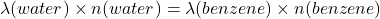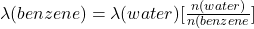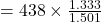## light of a certain frequency has a wavelength of 438 nm in water.What is the wavelength of this light in benzene​

Question

light of a certain frequency has a wavelength of 438 nm in water.What is the wavelength of this light in benzene​

in progress 0
5 months 2021-08-27T04:19:11+00:00 1 Answers 41 views 0

388.97 nm

Explanation:

The computation of the wavelength of this light in benzene is shown below:

As we know that

n (water) = 1.333

n (benzene) = 1.501And, the wavelength of water is 438 nmNow placing these values to the above formula

So,= 388.97 nm

We simply applied the above formula so that we can easily determine the wavelength of this light in benzene​ could come